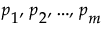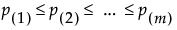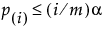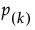All of the Response Screening plots involve p-values for tests conducted using the FDR technique described in Benjamini and Hochberg, 1995. See also Westfall et al. (2011).
 1 Conduct the usual hypothesis tests of interest, to obtain p-values.
 2 Rank the p-values from smallest to largest. Denote these by.
 3 Find the largest p-value for which. Suppose this first p-value is the kth largest,.
 4 Reject the k hypotheses associated with p-values less than or equal to.
The p-values adjusted for the false discovery rate, denoted, are computed as:
If a hypothesis has an FDR-adjusted p-value that falls below α, then it is rejected by the procedure.